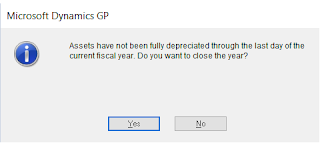It's occasionally encountered in Fixed Assets to see a negative net book value which is not quite logical since the Life to Date depreciation amount with the Remaining Appreciable amount should net to Zero. GP end users usually post questions on the Microsoft Dynamics GP Community about such cases almost every year especially when year end closing approaches. In this essence, the important question is why you might see "negative" net book value for some assets in Dynamics GP ?

The most common reason for such a case is to close the fixed asset year before all assets have been fully depreciation. Despite of the fact that the system usually pre-check whether all assets have been fully depreciated when the year end closing procedure is run, the end user can still ignore that warning and proceed with the closing procedure.

Here is the warning which pops up when running  year-end closing before depreciating all assets:Year-end closing Warning

Before getting into the resolution part, it's important to mention several important information related to the fixed asset details:
• Net Book Value is basically a calculated field which is a result of Cost Basis - LTD Depreciation Amount
• Cost Basis is the depreciable amount of the asset.
• LTD Depreciation Amount Displays the amount of depreciation calculated for this asset book since the date the asset was placed in service. The amount in this field includes the year-to-date depreciation amount.
In order to check whether you have any fixed assets with Negative Book Value, you may simply to to Microsoft Dynamics GP > Smart List > Fixed Assets > Fixed Assets Books On the smart list, you may filter by having Net Book Value <  0

On the SQL, you may simply run the blow query and it will retrieve list of all assets with a negative net book value:

SELECT [Asset ID],
Suf,
[Asset Description],
[Asset Class ID],
[Depreciated to Date],
[Cost Basis],
[YTD Depreciation Amount],
[LTD Depreciation Amount],
[Net Book Value],
[Cost Basis] - [LTD Depreciation Amount] -- NetBookValue_CalculatedField
FROM dbo.FixedAssetsBooks;

Resolution

The resolution is represented with simply "Resetting the Life" of the specific asset with the negative net book value. In order to do so, follow the steps below:

1. Go to Microsoft Dynamics GP > Tools > Routines > Fixed Assets > Depreciate One Asset
2. Pick the asset with the negative book value from the Asset ID look up, and specify the asset suffix ( usually it is 1, unless you use it for creating multiple assets)
3. Leave the Depreciation Target Date blank ( 00 00 0000 )
4. Select the  book for the fixed asset to be reset
5. Click on "Reset Life"
6. The above will recalculate the Life to Date depreciation up until the depreciate to date. It will create GL Journals in the "FA GL Posting"
7. Delete any GL Journals in the "FA GL Posting" for that specific aset

Here is the Depreciate One Asset ScreenDepreciate One Asset - Reset Life

Best Regards,